Topics: Symmetry 18 images tree :: Antiprism Examples and Visual Reference > Topics and Overviews > Topics: Symmetry
In This Album:

 Image List Thumbnail Page
Several programs have features involving symmetry:
• antiview, off2vrml, off2pov - display symmetry elements
• poly_kscope - repeats a model symmetrically, used to make compounds
• off_align - repeats a model symmetrically, used to augment polyhedra
• off_trans - aligns a model according to symmetry
• off_report - prints symmetry information for a model
• off_color - colours by symmetry orbit
The program option parameters are organised around the following ideas
Symmetry group
A set of (Euclidean) transformations that carry a polyhedron onto itself, described in general form using Schoenflies notation (see below) e.g. Oh, D3v.

Full symmetry group of a polyhedron
The set of all (Euclidean) transformations that carry a polyhedron onto itself.

Symmetry subgroup, or subsymmetry
A set of transformations from a symmetry group which, considered alone, also form a symmetry group, e.g. a cube has Oh symmetry and has a 3-fold axis corresponding to a C3v subgroup.

Symmetry orbit of an element
A set of equivalent elements, those elements that this element is carried on to by a symmetry or subsymmetry of the model.

Standard alignment of a symmetry
A symmetry group could be aligned anywhere in the coordinate system, but there are particular alignments that fit nicely with the coordinate axes, and these are used as the 'standard' alignments in Antiprism. They are a way of associating a symbol like D3v with a fixed set of transformations.

Conjugation subtype of a subsymmetry
This is an integer used to distinguish subgroups which are not carried onto each other (by conjugation) by the transformations of the parent symmetry group. For example a cube has a 2 fold axis through mid-edge and a 2-fold axis through a face centre. There is no symmetry of the cube that carries one onto the other and so they will have different subtype numbers. Geometrically, they look different in the cube.

Symmetry realignment
If you align a polyhedron with the standard set of symmetries for its full symmetry group there is often more than one distinct way to achieve this (a transformation not in the symmetry group that transforms the symmetry group onto itself). For example, if you align a cereal box-like cuboid naturally with the coordinate axes there are 6 possibilities i.e. the centres of the three rectangle types can lie on any of the axes, with 3x2x1 = 6. Possibilities for some polyhedra are infinite e.g. the symmetry group of a pyramid does not change when it is translated along its principal axis. The realignment is given by a series of colon separated numbers, the first number selects from a finite set of realignments, and the following numbers are decimals to control rotations and translations as follows:
• axial rotation: 1 number - degrees around principle axis
• full rotation: 3 numbers - degrees around x, y and z axes
• axial translation:i 1 number - distance to translate along principal axis
• plane translation: 2 numbers - distance to translate along two orthogonal directions in (mirror) plane
• full translation: 3 numbers - distance to translate along x, y and z axes

Schoenflies notation
Used to specify symmetry groups. The standard alignments have, preferentially, a centre (fixed point) on the origin a principal rotational axes on the z-axis, a dihedral axis on the x-axis, a mirror normal on the y-axis (except Cs has a mirror normal on the z-axis). The polyhedral symmetry types have a 3-fold axis on (1,1,1). In the following list of symbols, when a type contains 'n' this must be replaced by an integer (giving an n-fold axis), and for S this integer must be even.
• C1 - identity
• Cs - mirror
• Ci - inversion
• Cn - cyclic rotational
• Cnv - cyclic rotational with vertical mirror
• Cnh - cyclic rotational with horizontal mirror
• Dn - dihedral rotational
• Dnv - dihedral rotational with vertical mirror
• Dnh - dihedral rotational with horizontal mirror
• Sn - cyclic rotational (n/2-fold) with rotation-reflection
• T - tetrahedral rotational
• Td - full tetrahedral
• Th - tetrahedral with inversion (pyritohedral)
• O - octahedral rotational
• Oh - full octahedral
• I - icosahedral rotational
• Ih - full icosahedral
There are two symmetry reports. off_report -S s gives a general listing of the full symmetry, subsymmetries with numer of types, realignment possibilities and the axes with their number
```    off_report -S s rh_cubo
```
The subsymmetry and realignment possibilities indicate what will be valid in the symmetry options. There is also a list of orbits, with the total number of orbits for each element type, and the number of elements in each orbit.
```    off_report -C O rh_cubo
```
A value of 1 for verts, edges, faces indicates the polyhedron is repsepctively isogonal, isotoxal, isohedral. The orbits can also be calculated for a subgroup, using the -y option
```    off_report -y D2h -C O rh_cubo
off_report -y D2h,1 -C O rh_cubo
```
These are the same orbits that are used for colouring. The first number indicates the number of colours that will be used, and the second numbers are the number of elements having each colour
```    off_color -f S rh_cubo | antiview
off_color -f S,D2h rh_cubo | antiview
off_color -f S,D2h,1 rh_cubo | antiview
```

 thumbnails 1/1
 View symmetry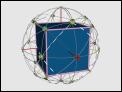Med  Lrg
 Kaleidoscope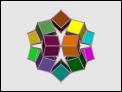Med  Lrg
 Excavate Full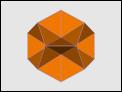Med  Lrg
 Augment Full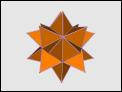Med  Lrg
 Augment Subsym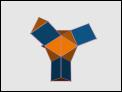Med  Lrg
 Align full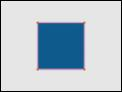Med  Lrg
 Align sub 0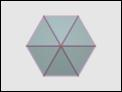Med  Lrg
 Align sub 1Med  Lrg
 Align sub 1 2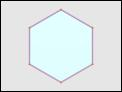Med  Lrg
 Align cuboidMed  Lrg
 Align with poly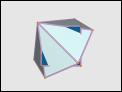Med  Lrg
 Align with polyMed  Lrg
 Align with polyMed  Lrg
 Align with polyMed  Lrg
 Align with sym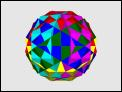Med  Lrg
 Colour Full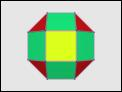Med  Lrg
 Colour Sub0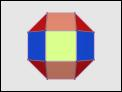Med  Lrg
 Colour Sub1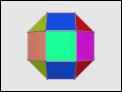Med  Lrg
 1